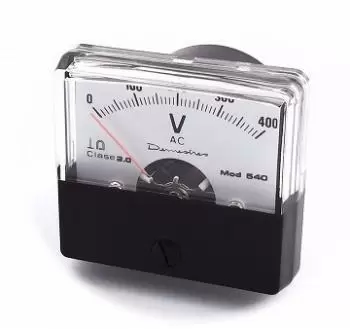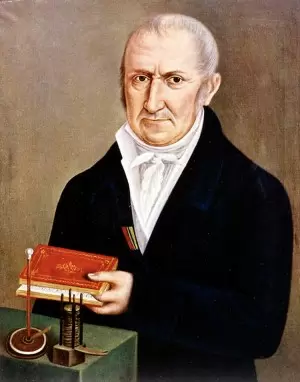# Definition of Volt - How Are Volts Measured?

The volt is the unit derived from the international system of measurements to measure the difference of electric potential, electric voltage or electromotive force. This unit refers to the amount of electrical energy required to move an electrical charge of one Coulomb through an electrical field. The symbol with which this unit is represented is V.The volt is defined as the current voltage that generates a current of one ampere that flows through an electrical conductor and consumes one watt of power.This unit receives its name in honor of the Italian physicist Alessandro Volta, inventor of the electric battery (or voltaic battery), the first chemical battery.

## How Are Volts Measured?

Voltage is measured using an instrument called a voltmeter. It works by connecting in parallel with the circuit component being measured and is configured depending on the type of voltage we want to measure.

The voltmeter must have a very high internal resistance to prevent circuit current from being shunted into the voltmeter and changing the measured voltage. Therefore, an ideal voltmeter would have infinite internal resistance.

There are two types of voltmeters: analog and digital.

• An analog voltmeter uses a needle and scale to display the measured voltage.

• A digital voltmeter uses a numeric display to show the measured voltage and is usually more accurate.

## Examples of Measurements

Here are some examples of volts in different situations:

1. A typical household plug provides a voltage of 120 volts alternating current (AC) in North America, while in other countries such as Europe, the voltage of a household outlet is 220-240 volts AC.

2. A typical electric car battery produces a voltage of 12 volts DC (direct current).

3. Energy efficient LED lights used in homes and buildings are often powered by 12 volts DC.

4. A typical mobile phone has a battery that produces between 3.7 and 4.2 volts DC.

5. A wind power generator produces a variable alternating current (AC) voltage, which is then converted to direct current (DC) for use in homes and buildings.

6. A car's electrical system, which includes the battery, alternator, and electronics, operates on a variety of voltages, including 12, 24, and 48 volts.

7. Solar panels produce voltages that vary depending on their size and design, but generally produce between 12 and 24 volts of direct current.

In general, voltages can vary widely depending on the application and the specific requirements of each device or electrical system.

## The Relationship Between the Volt and Electrical Energy

The volt and electrical energy are closely related because voltage affects the amount of electrical energy that flows through an electrical circuit. Electrical power is calculated by multiplying electrical power by time, and electrical power is calculated by multiplying voltage by electrical current.

The electrical power in watts (W) is equal to the number of volts (V) x amps (A).

Therefore, if the voltage is increased, the electrical power and electrical energy will also be increased, as long as the electrical current remains constant.

This relationship between voltage and current is also defined by Ohm's law. Ohm's law states that the potential difference V between two points on a conductor is proportional to the intensity of the current flowing through it.

Author:

Published: May 13, 2015
Last review: April 17, 2023